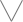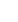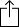C
D
E
F
G
A
B
Chord
Scale
3nps
CAGED
None
Notes
Degrees
None

1
2
3
4
5
6
7
8
9
10
11
12
13
14
15
16
17
18
19
20
21
22
23
24
E
B
G
D
A
E
F
F
G
G
A
B
B
C
C
D
E
E
F
F
G
G
A
B
B
C
C
D
E
E
C
C
D
E
E
F
F
G
G
A
B
B
C
C
D
E
E
F
F
G
G
A
B
B
G
A
B
B
C
C
D
E
E
F
F
G
G
A
B
B
C
C
D
E
E
F
F
G
E
E
F
F
G
G
A
B
B
C
C
D
E
E
F
F
G
G
A
B
B
C
C
D
B
B
C
C
D
E
E
F
F
G
G
A
B
B
C
C
D
E
E
F
F
G
G
A
F
F
G
G
A
B
B
C
C
D
E
E
F
F
G
G
A
B
B
C
C
D
E
E

# E Pentatonic for Guitar

E Pentatonic, sometimes called "E Major Pentatonic" is a pentatonic scale. Pentatonic scales have 5 notes, "penta" comes from the greek word "Pentateuch" which means 5.

The E Pentatonic scale is derived from the E Major scale by taking scale degree 1, 2, 3, 5 and 6 of the scale.

E
F
G
B
C
EW
W
m3
W
m3

# Metronome 60bpm• Options
• Player
Install fretastic on your iPhone:
tapand then
"Add to homescreen"